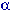### Conventional methods compared to

[dd. 2022|05|05 - 17h:58m (40s:451ms) v. 1.1 /  ]

### Analysis /operation

Conventional approach.

### Language analysis

Structure of language

Syntaxis:

Ill-formed
(Deletions, disorder/ fragmentation, overlaps)
≅ 0.6
Wellformed
(Complete, sound, coherent, delimited)
≅ 0.8

((1 -0.6 ) /0.8 =)
50%

Semantics:

Insufficiently interpretable
(Diffuse reference, qualification, manipulated meaning)
≅ 0.6
Reference-decidable
(Sensory /descriptive, sharp reference, stable denotations)
≅ 0.8

((1 -0.6 ) /0.8 =)
50%

Combined (chain-inference)

(0.5 *0.5 =)

### Logic

Logical formalization, valuation.
Consistency, validity, reduction, decidability.
'Dichotomous' logic: Conjunctive/exponential: Formal logic: Disjunctive/hyper-exponential:
Decision time Complexity

(2  poly(n) )

### O

(2  (2  poly(n) ) )
Combinatory explosion =EXPTIME =2-EXPTIME
n =2 :
4
16
(4 /16 =) ______25.000%

n =3 :
8
256
(8 /256 =) _______3.125%

n =4 :
16
65536
(16 /65536 =) ____0.024414%

'Average' ≅

(8 /256 = 1 /32 =)

### Statistical-causal analysis

Minimum proportion of variance explained required for reliable prediction by correlation measured (=0.05).
Induction: Sample (N=100) ' Significance'
(1 -0.000095 =) 0.999905

Prediction : Population to new sample avarage
(Extrapolation)

Predictive power
(decile =0.1)
(1 -0.995898 =)
____0.004102

(1 /__243.783520 =)
____0.410200%

Deduction : Population to Individual
('N=1' prediction)

Individual
(decile =0.1, ε =0.05)
(1 -0.999711 =)
____0.000288

(1 /___14.193771 =) ____7.045343%

Combined (Chain-inference)
(1 /_3460.207612 =)

### Logical-causal analysis

Causale hypothese: Observatie naar theorie.
Conditional probabilities of causal variants contingent under correlation measured.

Disjunct effect:
(1 -0.875 =) 0.125
(1 -0 =) 1
(1 /8 =) ______12.500%

Common cause:
(1 -0.75 =) 0.25
(1 -0 =) 1
(1 /4 =) ______25.000%

Disjunct-conjunct complex cause:
(1 -0.8125 =) 0.1875
(1 -0 =) 1
(1 /5.333333 =) ______18.750%

Intermediating disjunct cause:
(1 -0.75 =) 0.25
(1 -0 =) 1
(1 /4 =) ______25.000%

Combined
contingency
(1 -0.90625 =) 0.09375
(1 -0 =) 1
(1 /10.666666 =)

### Causal inference

(overall)
(____0.000288
*____0.093750 =)

### Psychology

Deterministic- fysicalistic model ('bio-robot model')
Integrated holistic model

Factor model complexity: ≅
Four factor model
(stimulus, nature, nurture, response)
≅ 4
Ten Factor Model
(stimulus, genetics, perception, body state, memory, processing, consciousness, emotion, choice, response)
≅ 10

Average ≅
(4 /10 =)

### (product)   ≅

(1 /______11810841.245 =)